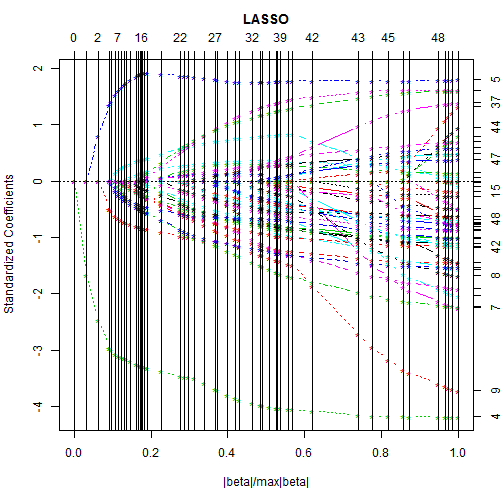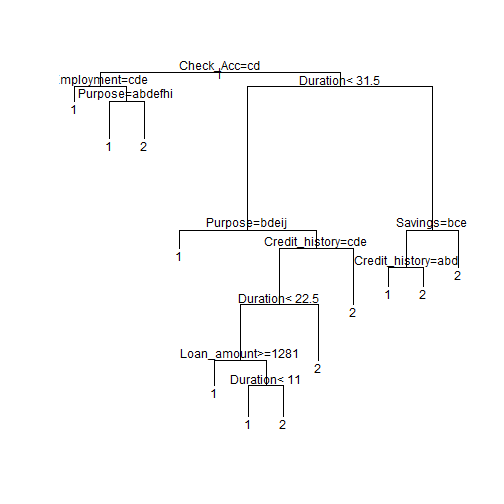# Prediction methods analysis with the German Credit Data set

Tao Lin (Richie)
12/29/2015

### Dataset introduction

This is a dataset that been widely used for machine learning practice. Here are some breif introduction to this dataset:

• There are 1000 observations in this dataset.
• 20 independent variables are there in the dataset, the dependent variable the evaluation of client's current credit status.
• Data in this dataset have been replaced with code for the privacy concerns.

### Prediction methods introduction

For this dataset, I am going to use four commonly used methods to build the machine learning model for our prediction.

• Logistic Regression
• LASSO (least absolute shrinkage and selection operator)
• Classification Tree
• Random Forest

### Dataset decryption and cleaning

The dataset was encrypted, we need to decrypt it with the code book.

Before

summary(df1)[,c(1,3,4)]

 Check_Acc   Credit_history    Purpose
"A11:274  " "A30: 40  "    "A43    :280  "
"A12:269  " "A31: 49  "    "A40    :234  "
"A13: 63  " "A32:530  "    "A42    :181  "
"A14:394  " "A33: 88  "    "A41    :103  "
NA          "A34:293  "    "A49    : 97  "
NA          NA             "A46    : 50  "
NA          NA             "(Other): 55  "


### Dataset decryption and cleaning

After

summary(df1)[,c(1,3,4)]

                  Check_Acc
"      ... <    0 DM   :274  "
" 0 <= ... <  200 DM   :269  "
"      ... >= 200 DM / : 63  "
" no checking account  :394  "
NA
NA
NA
Credit_history
" no credits taken/                       : 40  "
" all credits at this bank paid back duly : 49  "
" existing credits paid back duly till now:530  "
" delay in paying off in the past         : 88  "
" critical account/                       :293  "
NA
NA
Purpose
" domestic appliances :280  "
" car (new)           :234  "
" car (used)          :103  "
" others              : 97  "
" retraining          : 50  "
"(Other)              : 55  "


### Dataset preperation for model building

We are going to divide the dataset into 0.7:0.3 for training and testing the model. For the logistic regression, we also need to transform the data frame with factors into the matrix with biominal value.

mat1 <- model.matrix(Credit ~ . , data = df1  )
n<- dim(df1)

set.seed(1234)
train<- sample(1:n , 0.7*n)
xtrain<- mat1[train,]
xtest<- mat1[-train,]

ytrain<- df1$Credit[train] ytest<- df1$Credit[-train]


### Logistic Regression

Build the logistic Regression model.

m1 <- glm(Credit ~ . , family = binomial, data= data.frame(Credit= ytrain, xtrain))


### Logistic Regression

Key Variables for the regression model.

sig.var<- summary(m1)$coeff[-1,4] <0.01 names(sig.var)[sig.var == T]   "Check_Acc.no.checking.account"  "Duration"  "Credit_history.critical.account.."  "Purpose.car..used.."  "Purpose.radio.television."  "Purpose.domestic.appliances."  "Savings................1000.DM"  "Savings...unknown..no.savings.account"  "Rate_of_income"  ### Logistic Regression Predit outcome with Logistic Regression model, then use the test dataset to evaluate the model. pred1<- predict.glm(m1,newdata = data.frame(ytest,xtest), type = "response") result1<- table(ytest, floor(pred1+1.5)) result1   ytest 1 2 1 176 25 2 51 48  error1<- sum(result1[1,2], result1[2,1])/sum(result1) error1   0.2533333  ### LASSO LASSO is a useful method to generate logistic regression with seleted key variables. lasso<- lars(x= mat1, y= as.numeric(df1$Credit), trace = T)


Plot the LASSO variables selection procedure### LASSO

Predit outcome with model generated by LASSO, then use the test dataset to evaluate the model

result2


ytest   1   2
1 162  39
2  34  65

error2

 0.2433333


### Classifiation Tree

Build the Classification Tree with rpart function.

m3.tree<- rpart(Credit ~ . , data = df1[train,], method= "class")
printcp(m3.tree)


Classification tree:
rpart(formula = Credit ~ ., data = df1[train, ], method = "class")

Variables actually used in tree construction:
 Check_Acc      Credit_history Duration       Employment
 Loan_amount    Purpose        Savings

Root node error: 201/700 = 0.28714

n= 700

CP nsplit rel error  xerror     xstd
1 0.057214      0   1.00000 1.00000 0.059553
2 0.034826      2   0.88557 1.00498 0.059641
3 0.029851      5   0.78109 1.01990 0.059901
4 0.022388      6   0.75124 0.96020 0.058822
5 0.014925      8   0.70647 0.96517 0.058916
6 0.010000     11   0.66169 1.02985 0.060071


### Classifiation Tree

Plot the classification Tree.### Classifiation Tree

Use the tre model to predict and evaluate.

result3


ytest   0   1
1 185  16
2  58  41

error3

 0.2466667


### Random Forest

Random forests is a notion of the general technique of random decision forests that are an ensemble learning method for classification.

Call random forest classification function:

m4.rf<- randomForest(Credit ~ . , data = df1[train,], method= "class", ntree = 500, mtry = 10)


### Random Forest

Result:


Call:
randomForest(formula = Credit ~ ., data = df1[train, ], method = "class",      ntree = 500, mtry = 10)
Type of random forest: classification
Number of trees: 500
No. of variables tried at each split: 10

OOB estimate of  error rate: 23%
Confusion matrix:
1  2 class.error
1 457 42  0.08416834
2 119 82  0.59203980


### Conclusion

Error rate of each method:

• Logistic Regression: 25.33%
• LASSO: 24.33%
• Classification Tree: 24.67%
• Random Forest: 23.71%